Ask your WordPress questions! Pay money and get answers fast!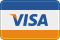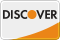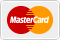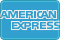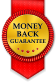# Undefined index: std and id in my theme WordPress

• SOLVED

I have an issue when I activate WP_DEBUG.

The issue apparently is on this line...

"\$defaults[\$value['id']] = \$value['std'];"

Here's the code...

``` if (\$value['type'] == 'multicheck'){ if (is_array(\$value['std'])){ foreach(\$value['std'] as \$i=>\$key){ \$defaults[\$value['id']][\$key] = true; } } else { \$defaults[\$value['id']][\$value['std']] = true; } } else { \$defaults[\$value['id']] = \$value['std']; } ```

Any ideas?

2011-04-25

```if ( isset( \$value['type'] ) && isset( \$value['id'] ) && isset( \$value['std'] ) && 'multicheck' == \$value['type'] ) { if ( is_array( \$value['std'] ) ){ foreach( \$value['std'] as \$i => \$key ){ \$defaults[\$value['id']][\$key] = true; } } else { \$defaults[\$value['id']][\$value['std']] = true; } } else if ( isset( \$value['id'] ) && isset( \$value['std'] ) ) { \$defaults[\$value['id']] = \$value['std']; }```